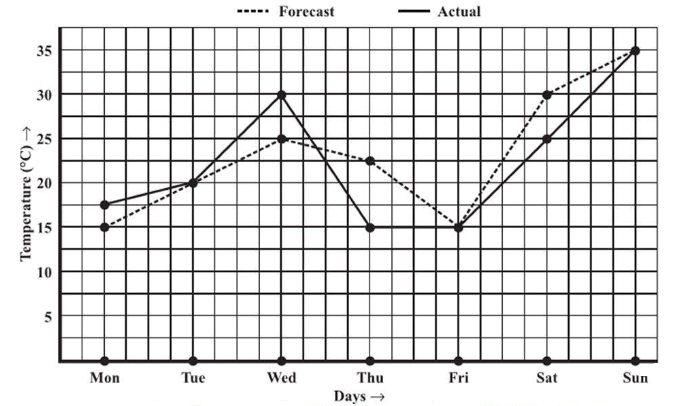# Ex.15.1 Q4 Introduction to Graphs Solutions - NCERT Maths Class 8

## Question

The following graph shows the temperature forecast and the actual temperature for each day of a week.

(a) On which days was the forecast temperature the same as the actual temperature?

(b) What was the maximum forecast temperature during the week?

(c) What was the minimum actual temperature during the week?

(d) On which day did the actual temperature differ the most from the forecast temperature?Video Solution
Introduction To Graphs
Ex 15.1 | Question 4

## Text Solution

Reasoning:

Horizontal axis represents ‘Days’ and vertical represents ‘Temperature’ in $$\rm{C\;^\circ}$$

We can find the value of ‘Temperature’ on vertical axis according to the given value of ‘Days’ on the horizontal axis value or vice versa.

Steps:

(a) The forecast temperature was same as the actual temperature on Tuesday, Friday, and Sunday.

(b) The maximum forecast temperature during the week was $$35^\circ \rm{C.}$$

(c) The minimum actual temperature during the week was $$15^\circ\rm{C.}$$

(d) The actual temperature differs the most from the forecast temperature on Thursday.

Learn from the best math teachers and top your exams

• Live one on one classroom and doubt clearing
• Practice worksheets in and after class for conceptual clarity
• Personalized curriculum to keep up with school